### The Three Assumptions of ANOVA

1. Assumption of independence

ANOVA assumes that the observations are random and that the samples taken from the populations are independent of each other. One event should not depend on another; that is, the value of one observation should not be related to any other observation.

Independence of observations can only be achieved if you have set your experiment up correctly. There is no way to use the study’s data to test whether independence has been achieved; rather, independence is achieved by correctly randomising sample selection. If the observations are not independent, then the one-way ANOVA is an inappropriate statistic.

1. Assumption of homogeneity of variance

ANOVA assumes that the variances of the distributions in the populations are equal. Remember, the purpose of the ANOVA test is to determine the plausability of the null hypothesis, where the null hypothesis says that all observations come from the same underlying group with the same degree of variability. Therefore, if the variances of each group differ from the outset, then the null hypothesis will be rejected (within certain limits) and thus there is no point in using ANOVA in the first place.

1. Assumption of normality

ANOVA is based on the F-statistic, where the F-statistic requires that the dependent variable is normally distributed in each group. Thus, ANOVA requires that the dependent variable is normally distributed in each group.

### Testing the Three Assumptions of ANOVA

We will use the same data that was used in the one-way ANOVA tutorial; i.e., the vitamin C concentrations of turnip leaves after having one of four fertilisers applied (A, B, C or D), where there are 8 leaves in each fertiliser group.

vitc <- read.csv("vitc_data.csv")

Now let us perform the ANOVA just like we did in the one-way ANOVA tutorial. We want to model the vitamin C concentrations (vit) on the fertiliser groupings (fert).

vitc_anova <- aov(vit ~ fert, data=vitc)
summary(vitc_anova)
##             Df Sum Sq Mean Sq F value   Pr(>F)
## fert         3  577.4  192.47   19.38 5.33e-07 ***
## Residuals   28  278.2    9.93
## ---
## Signif. codes:  0 '***' 0.001 '**' 0.01 '*' 0.05 '.' 0.1 ' ' 1
1. Assumption of independence

There is no way to test for independence of observations. This assumption can only be satisfied by correctly randomising your experimental design.

1. Assumption of homogeneity of variance
1. Bartlett’s test

Bartlett’s test tests the null hypothesis that the group variances are equal against the alternative hypothesis that the group variances are not equal. Bartlett’s test should be used when the data is normal and Levene’s test should be used when the data is non-normal - where Bartlett’s test is the more powerful of the two.

bartlett.test(vit ~ fert, data=vitc)
##
##  Bartlett test of homogeneity of variances
##
## data:  vit by fert
## Bartlett's K-squared = 1.3456, df = 3, p-value = 0.7183

Since the p-value is over 0.05, we fail to reject the null hypothesis and thus accept homogeneity of variances. However, let’s say the p-value was under 0.05; in this case, we could only conclude that at least one of the group variances was significantly different to the other group variances - but we wouldn’t know which group. Therefore it is always a good idea to graph the residuals in order to find out which group/s has the significantly different variance.

1. Boxplot

A quick way to get an idea about the variability within each group is to use a boxplot.

boxplot(vit ~ fert, xlab="Fertiliser group", ylab="Vitamin C concentration (%)", las=1, data=vitc)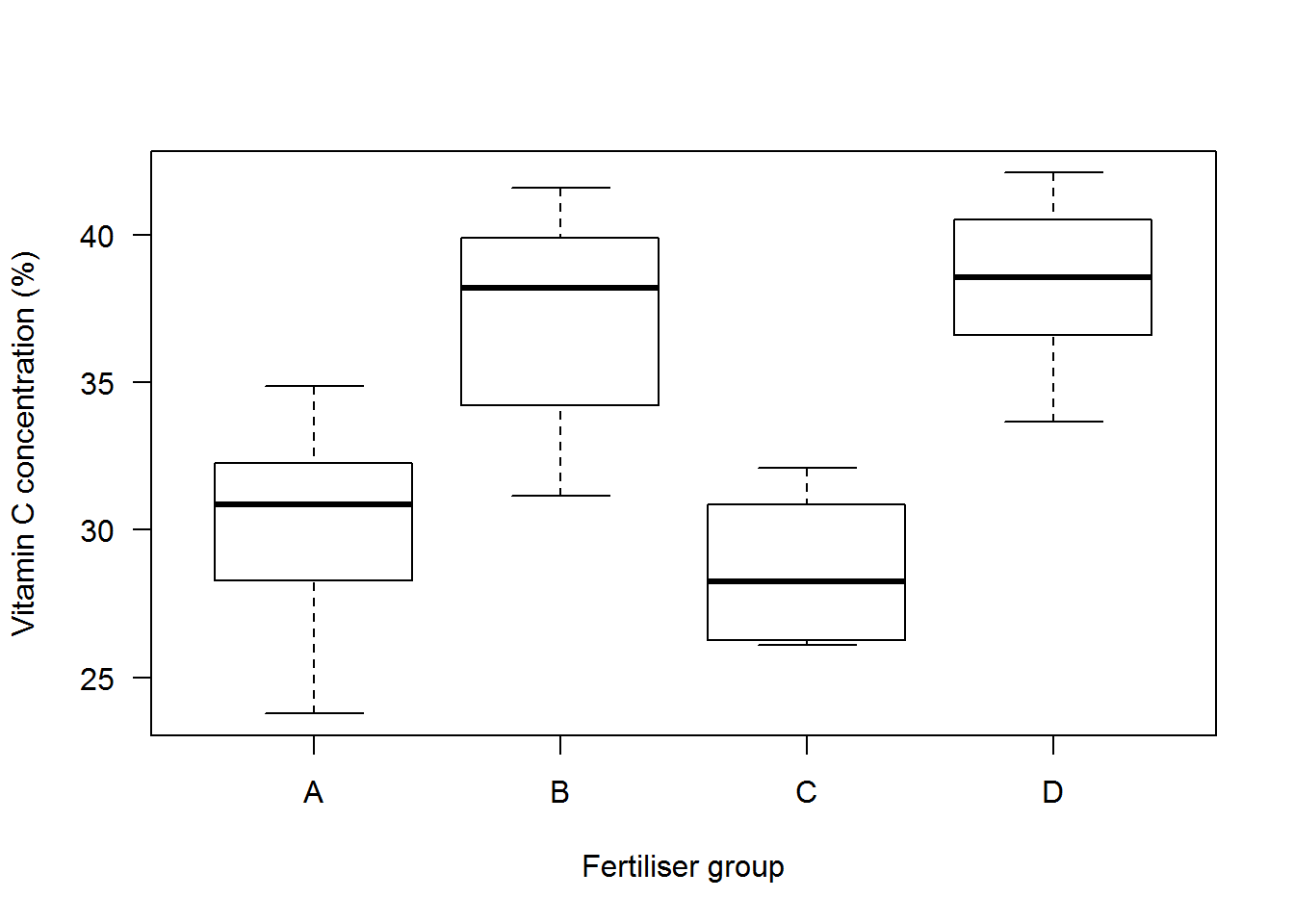The variability within each group is represented by the vertical size of each box; i.e., the interquartile range (IQR). The boxplot shows that the variability is roughly equal for each group. Let’s look at some more ways to test the homogeneity of variance assumption.

1. Residuals vs fitted Values

R has several inbuilt diagnostic tools that test the ANOVA assumptions. We can access these tools by plotting the output of our ANOVA test (i.e. vitc_anova).

plot(vitc_anova,1, las=1)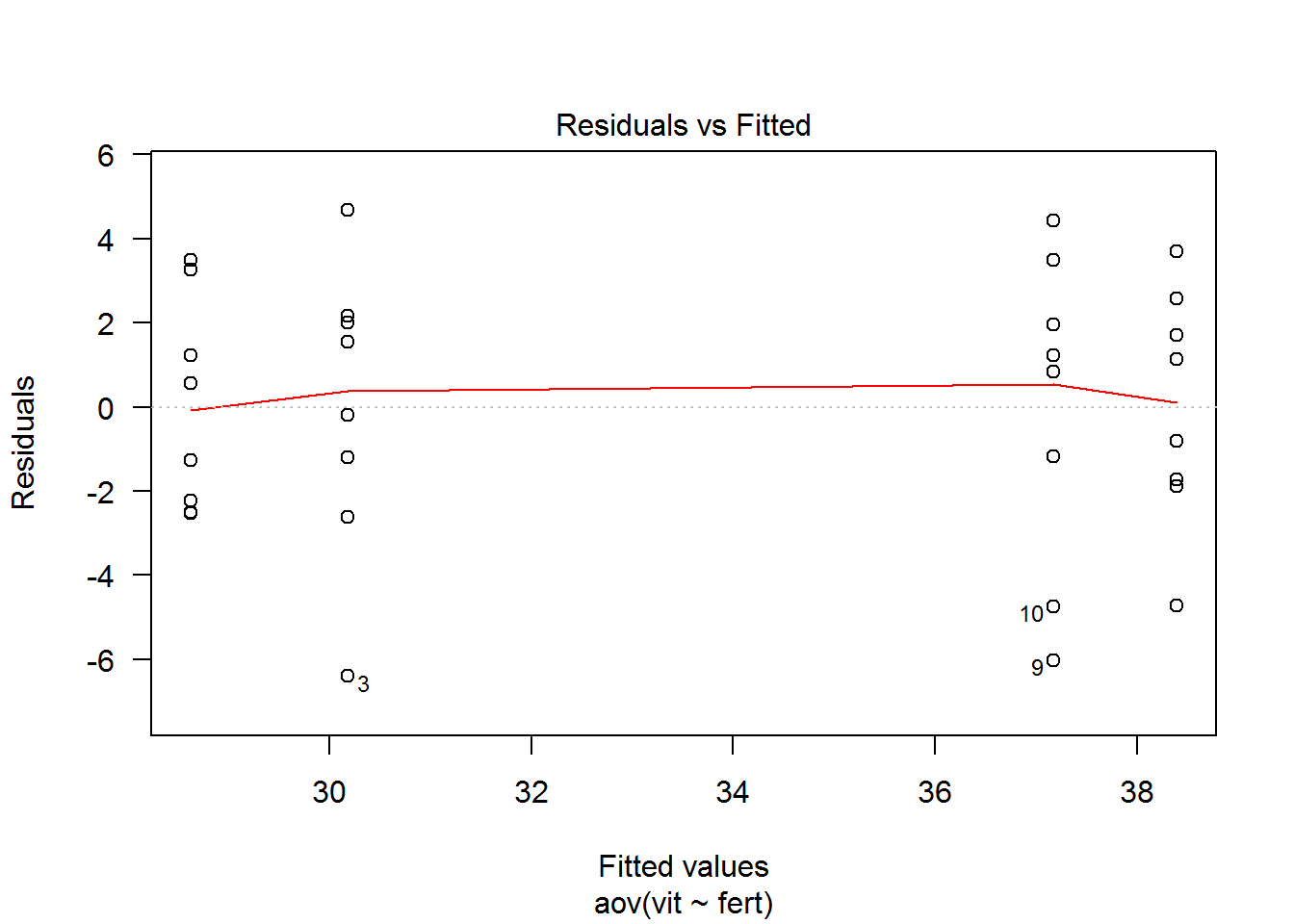This plot shows the residuals (errors) on the y-axis and the fitted values (predicted values) on the x-axis. If the variance of each group is equal, the plot should show no pattern; in other words, the points should look like a cloud of random points. The plot shows that the variances are approximately homogenous since the residuals are distributed approximately equally above and below zero.

If the red line is flat, then the relationship between the independent and dependent variables is linear. However, linearity is not an assumption of ANOVA so it will not be discussed here (but it will be discussed in the linear regression tutorial).

Another way we could have constructed the previous plot is to manually extract the residuals and the fitted values from the ANOVA result and plot them.

attributes(vitc_anova)
## $names ##  "coefficients" "residuals" "effects" "rank" ##  "fitted.values" "assign" "qr" "df.residual" ##  "contrasts" "xlevels" "call" "terms" ##  "model" ## ##$class
##  "aov" "lm"

We can see that “residuals” and “fitted.values” can be extracted from our ANOVA output. We can then plot them like so:

plot(vitc_anova$fitted.values, vitc_anova$residuals)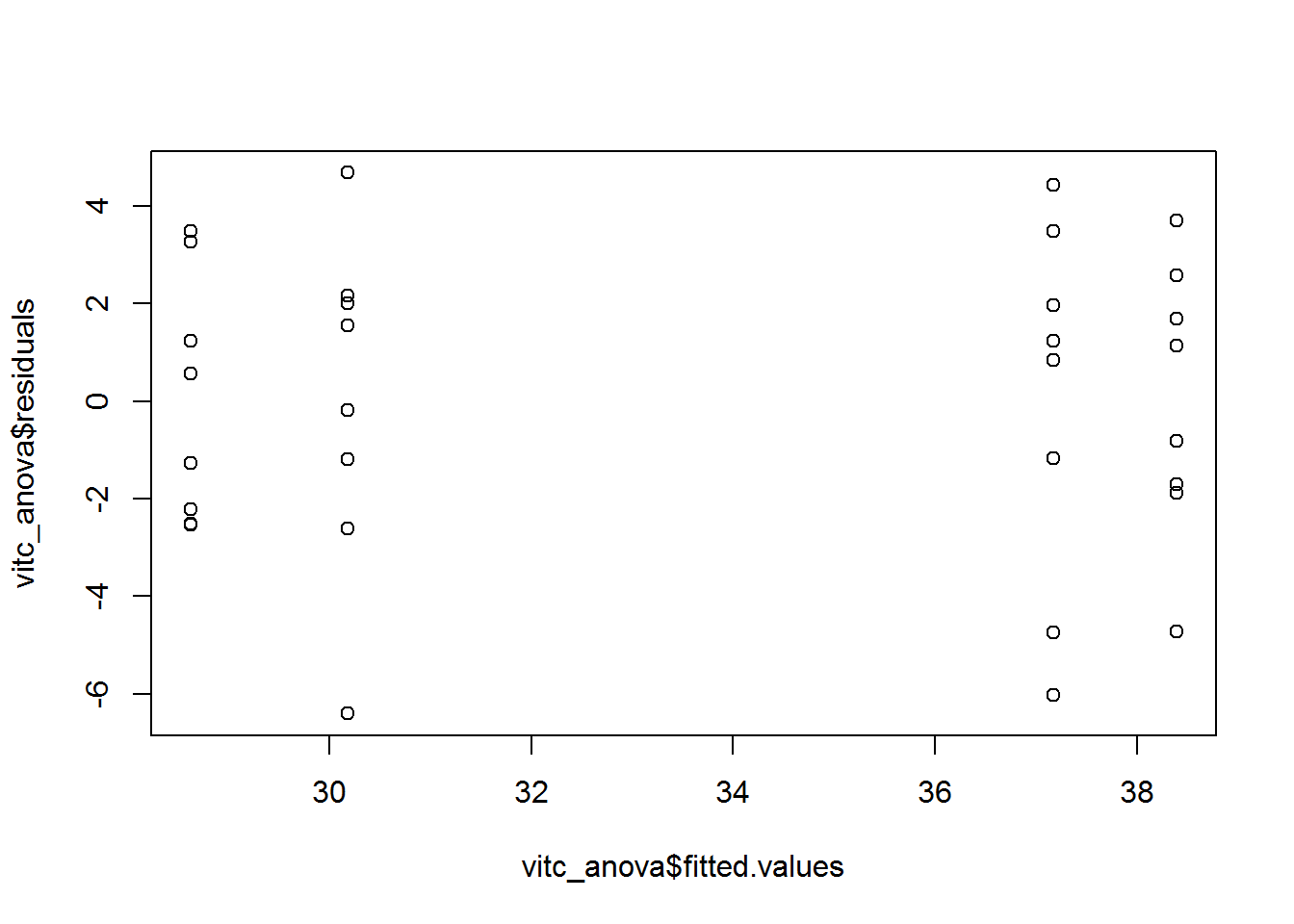1. Standardised residuals vs fitted values

Let’s look at another test that will help us test the homogeneity of variance assumption. This time we will divide each residual by its standard deviation; that is, each residual is made to have a standard deviation of 1. The standard deviation for residuals can vary a great deal from observation to observation so it is a good idea to standardise the residuals in order to allow easier comparisons. Standardised residuals are just scaled versions of the unstandardised residuals - and thus contain all the same information - so generally there is no reason to use unstandardised residuals in a diagnostic plot.

plot(vitc_anova,3)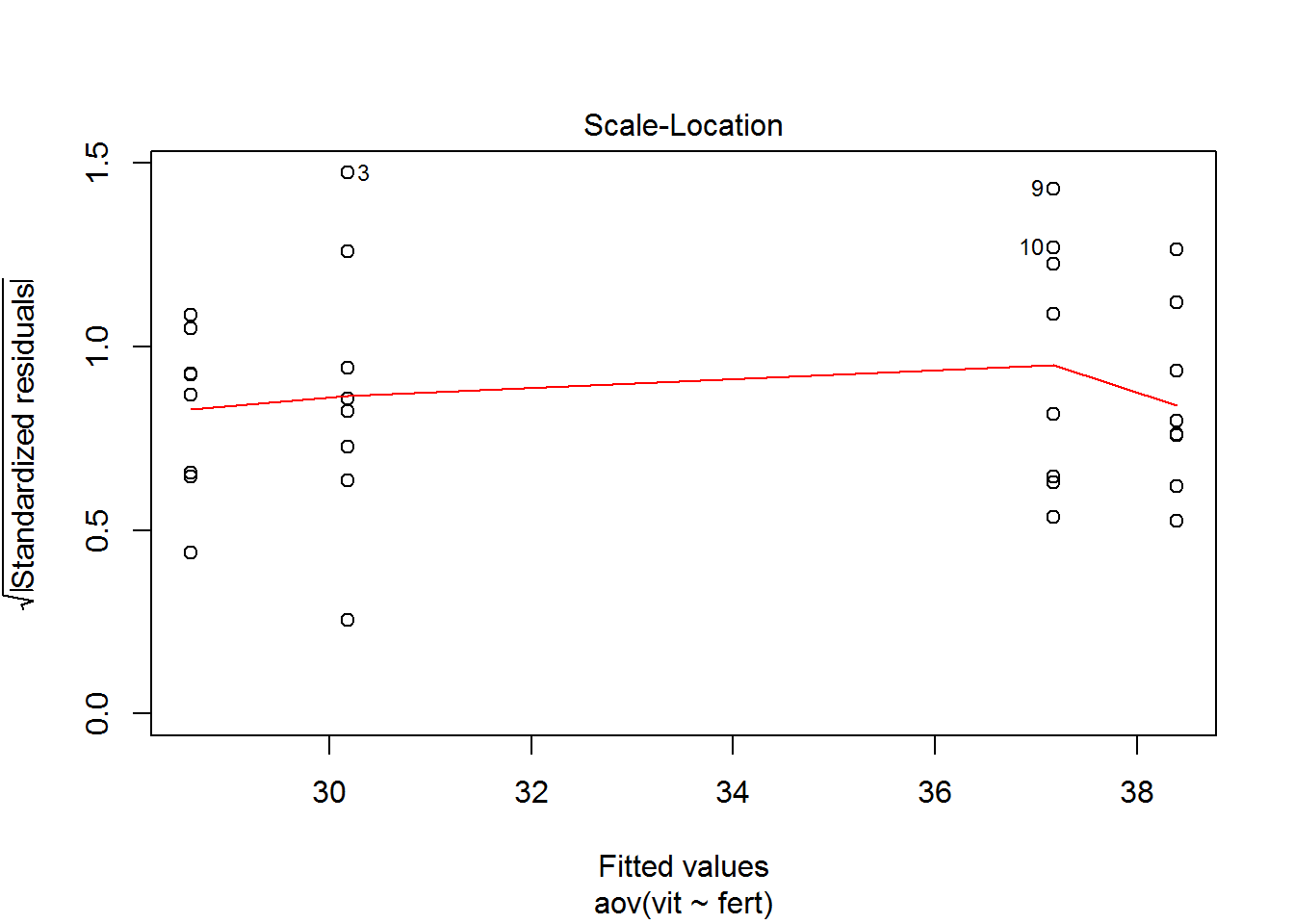Values above +2.5 or below -2.5 may be considered outliers. That is, values more than 2.5 standard deviations away from the mean may be considered outliers. However, this is just a rule of thumb. In a future lesson, we will investigate some more in-depth methods of detecting outliers like investigating concepts like ‘cook’s distances’, ‘leverage points’, and ‘influential points’.

1. Assumption of Normality
1. The Shapiro-Wilk test

The Shapiro-Wilk test tests the null hypothesis that the samples come from a normal distribution against the alternative hypothesis that the samples do not come from a normal distribution. Bartlett’s test should be used when the data is normal and Levene’s test should be used when the data is non-normal - where Bartlett’s test is the more powerful of the two.

shapiro.test(vitc$vit) ## ## Shapiro-Wilk normality test ## ## data: vitc$vit
## W = 0.95687, p-value = 0.2252

Since the p-value is over 0.05, we fail to reject the null hypothesis that the sample comes from a normal distribution. However, that is not to say that the data is indeed normal; to reiterate, accepting the alternative hypothesis does not necessarily follow from rejecting the null hypothesis. For this reason, the Shapiro-Wilk test is rarely used to detect normality since graphical representations are so much more useful. Furthermore, the Shapiro-Wilk test is sensitive to sample size. For small samples, even big departures from normality are not detected while for large samples small deviations from normality will lead to the null hypothesis being rejected.

1. Histograms

Histograms are a great first step into finding out the shape of a distribution.

hist(vitc$vit)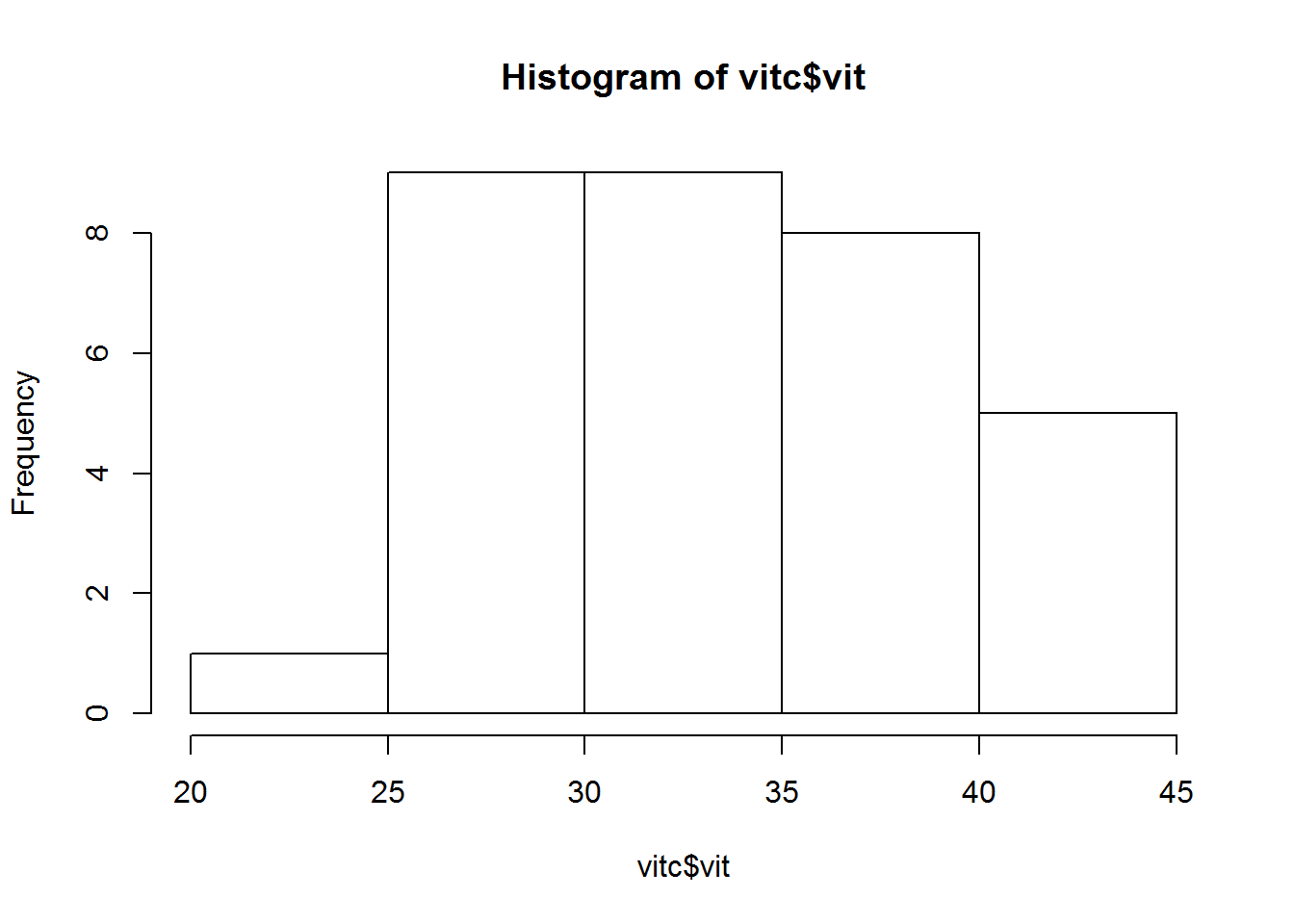Since the sample size is quite small, our histogram is not giving us a particularly clear picture. One could hesitantly say that the distribution looks normal, but we must gather more information. 1. QQ-plot The quantile-quantile plot or q-q plot plots the values as if they came from a normal distribution (the theoretically expected values) against the real values. The result is a graph that shows how far the real values stray from normality. qqnorm(vitc_anova$residuals)
qqline(vitc_anova\$residuals)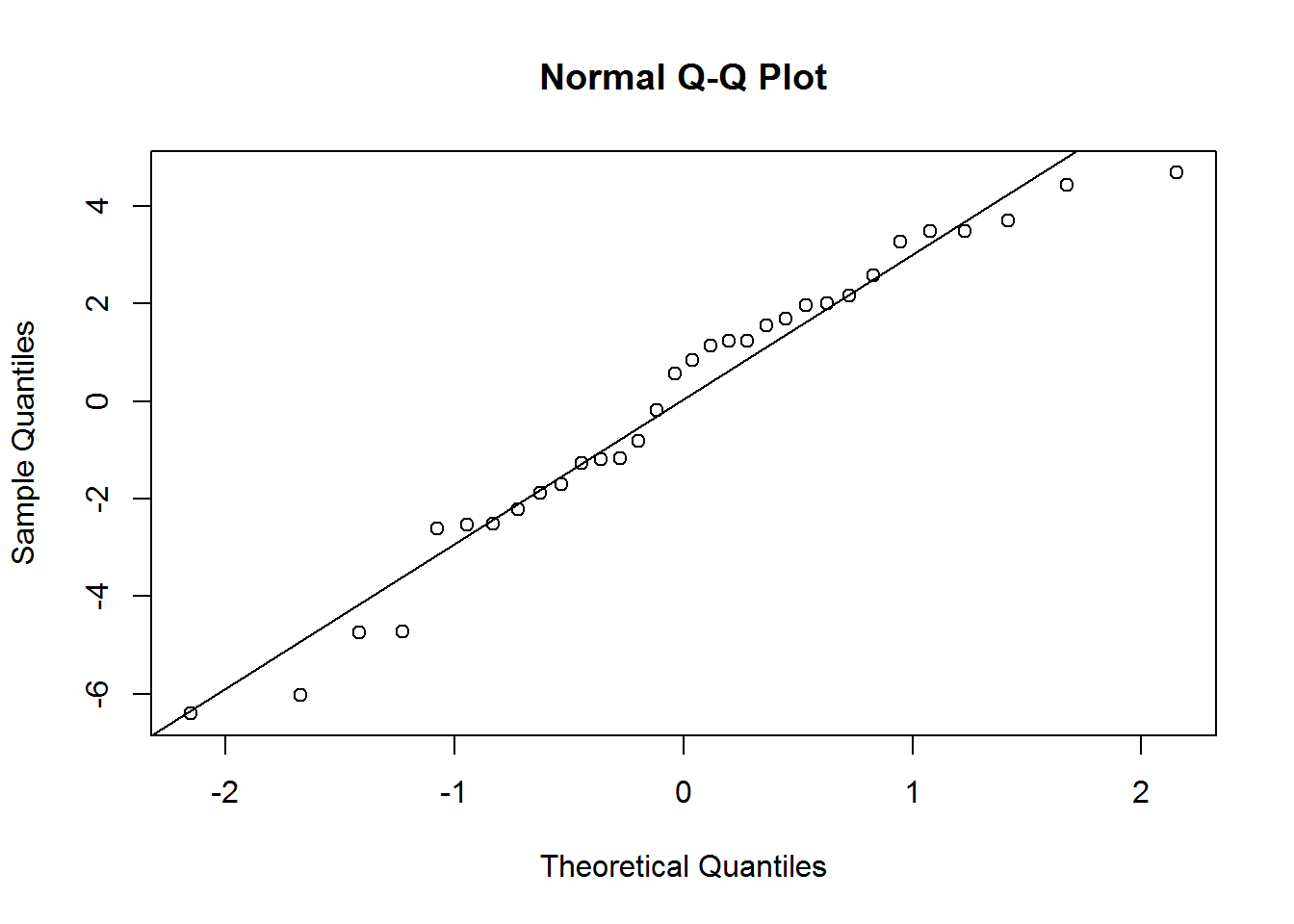The plot shows that the dependent variable is approximately normal, since the real observations approximately line-up with the theoretically-derived normal values.

### Conclusion

We have demonstrated homogeneity of variance and normality, thus one-way ANOVA is a valid test to determine any significant difference between group means (also assuming our study has been set-up ensure our observations are independent of each other).